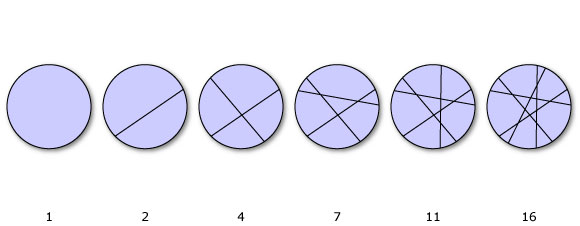## Central polygonal numbers

#### Rule

Each term is: Multiply by the next smaller number, divide by two, and add one

Known as: Central polygonal numbersThe maximum number of pieces of a circular pizza you can make with n straight slices.

#### The first 50 terms (starting from n=1) are:

1 2 4 7 11 16 22 29 37 46 56 67 79 92 106 121 137 154 172 191 211 232 254 277 301 326 352 379 407 436 466 497 529 562 596 631 667 704 742 781 821 862 904 947 991 1036 1082 1129 1177 1226

#### Example

To get the value for n=11:

• Multiply by the next smaller number, giving 110.
• Divide by two, giving 55.